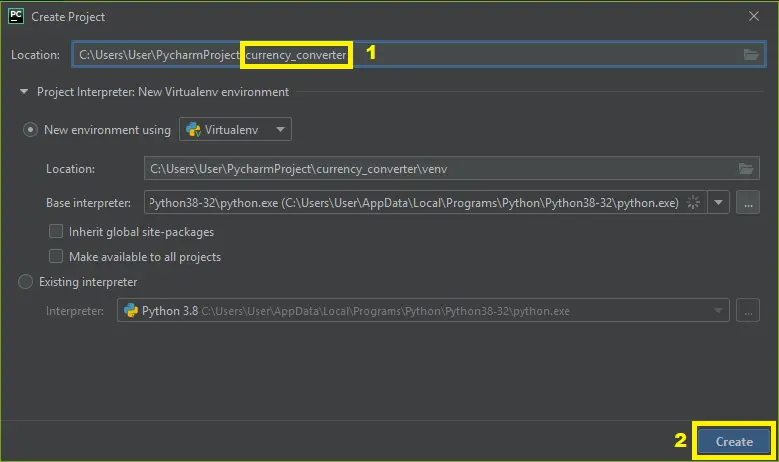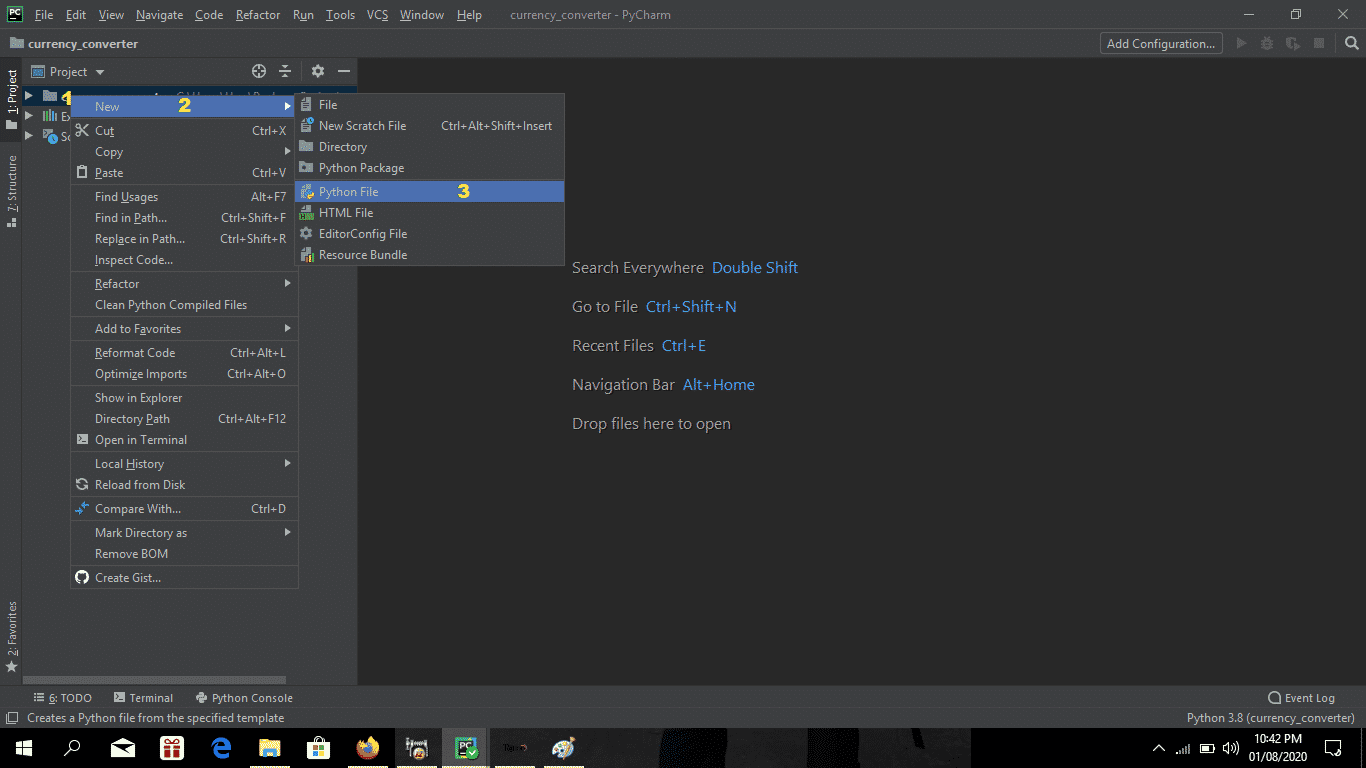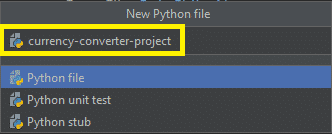# Currency Conversion Code in Python With Source Code

The Currency Conversion Code in Python develop an exciting python Program project through which you can convert currencies exchange rates. This article contains the Python Currency Converter Script (currency-converter-project.py).

## Currency Converter In Python : Project Information

Python Currency Converter is an app intermediate level python project which will boost your confidence. For a user interface, we can use Currency Converter Python Tkinter API. It includes also a downloadable Python Currency Converter Code for free.

To start creating this project Currency Conversion rates In Python, make sure that you have PyCharm IDE installed in you computer.

## Steps on how to create a Currency Conversion Code in PythonWith Source Code

Currency Conversion Code in Python With Source Code

• Step 1: Create a project name.

First open Pycharm IDE and then create a “project name” after creating a project name click the “create” button.• Step 2: Create a python file.

Second after creating a project name, “right click” your project name and then click “new” after that click the “python file“.• Step 3: Name your python file.

Third after creating a python file, Name your python file after that click “enter“.• Step 4: The actual code.

You are free to copy the code given below and download the full source code below.

The Code Given Below Is For Importing Modules.

``````import requests
from tkinter import *
import tkinter as tk
from tkinter import ttk``````

In this codes which is importing all the modules.

The Code Given Below Is For The Real time Currency Converter Class

``````class RealTimeCurrencyConverter():
def __init__(self,url):
self.data = requests.get(url).json()
self.currencies = self.data['rates']

def convert(self, from_currency, to_currency, amount):
initial_amount = amount
if from_currency != 'USD' :
amount = amount / self.currencies[from_currency]

# limiting the precision to 4 decimal places
amount = round(amount * self.currencies[to_currency], 4)
return amount``````

This module which is the module for the real time currency converter class.

The Code Given Below Is For Performing The Currency Conversion

`````` def perform(self):
amount = float(self.amount_field.get())
from_curr = self.from_currency_variable.get()
to_curr = self.to_currency_variable.get()

converted_amount = self.currency_converter.convert(from_curr,to_curr,amount)
converted_amount = round(converted_amount, 2)

self.converted_amount_field_label.config(text = str(converted_amount))

def restrictNumberOnly(self, action, string):
regex = re.compile(r"[0-9,]*?(\.)?[0-9,]*\$")
result = regex.match(string)
return (string == "" or (string.count('.') <= 1 and result is not None))``````

This module which is performing the currency conversion the you’ve select.

Complete Source Code

``````# # Python Project on Currency Converter

import requests
from tkinter import *
import tkinter as tk
from tkinter import ttk

class RealTimeCurrencyConverter():
def __init__(self,url):
self.data = requests.get(url).json()
self.currencies = self.data['rates']

def convert(self, from_currency, to_currency, amount):
initial_amount = amount
if from_currency != 'USD' :
amount = amount / self.currencies[from_currency]

# limiting the precision to 4 decimal places
amount = round(amount * self.currencies[to_currency], 4)
return amount

class App(tk.Tk):

def __init__(self, converter):
tk.Tk.__init__(self)
self.title = 'Currency Converter'
self.currency_converter = converter

#self.configure(background = 'blue')
self.geometry("530x200")

# Label
self.intro_label = Label(self, text = 'IT SOURCECODE Real Time Currency Converter',  fg = 'black',bg='blue', relief = tk.RAISED, borderwidth = 3)
self.intro_label.config(font = ('Courier',15,'bold'))

self.date_label = Label(self, text = f" Date : {self.currency_converter.data['date']}", relief = tk.GROOVE, borderwidth = 5)

self.intro_label.place(x = 10 , y = 5)
self.date_label.place(x = 200, y= 50)

# Entry box
valid = (self.register(self.restrictNumberOnly), '%d', '%P')
self.amount_field = Entry(self,bd = 3, relief = tk.RIDGE, justify = tk.CENTER,validate='key', validatecommand=valid)
self.converted_amount_field_label = Label(self, text = '', fg = 'black', bg = 'white', relief = tk.RIDGE, justify = tk.CENTER, width = 17, borderwidth = 3)

# dropdown
self.from_currency_variable = StringVar(self)
self.from_currency_variable.set("INR") # default value
self.to_currency_variable = StringVar(self)
self.to_currency_variable.set("USD") # default value

font = ("Courier", 12, "bold")
self.from_currency_dropdown = ttk.Combobox(self, textvariable=self.from_currency_variable,values=list(self.currency_converter.currencies.keys()), font = font, state = 'readonly', width = 12, justify = tk.CENTER)
self.to_currency_dropdown = ttk.Combobox(self, textvariable=self.to_currency_variable,values=list(self.currency_converter.currencies.keys()), font = font, state = 'readonly', width = 12, justify = tk.CENTER)

# placing
self.from_currency_dropdown.place(x = 30, y= 120)
self.amount_field.place(x = 36, y = 150)
self.to_currency_dropdown.place(x = 340, y= 120)
#self.converted_amount_field.place(x = 346, y = 150)
self.converted_amount_field_label.place(x = 346, y = 150)

# Convert button
self.convert_button = Button(self, text = "Convert", fg = "black", bg = "green", command = self.perform)
self.convert_button.config(font=('Courier', 10, 'bold'))
self.convert_button.place(x = 225, y = 135)

def perform(self):
amount = float(self.amount_field.get())
from_curr = self.from_currency_variable.get()
to_curr = self.to_currency_variable.get()

converted_amount = self.currency_converter.convert(from_curr,to_curr,amount)
converted_amount = round(converted_amount, 2)

self.converted_amount_field_label.config(text = str(converted_amount))

def restrictNumberOnly(self, action, string):
regex = re.compile(r"[0-9,]*?(\.)?[0-9,]*\$")
result = regex.match(string)
return (string == "" or (string.count('.') <= 1 and result is not None))

if __name__ == '__main__':
url = 'https://api.exchangerate-api.com/v4/latest/USD'
converter = RealTimeCurrencyConverter(url)

App(converter)
mainloop()

``````

## Summary

In this article, we labored at the Python undertaking to construct a Currency Converter.

I wish you discovered new matters and loved constructing this thrilling Python undertaking. Share the item on social media together along with your pals and colleagues.

## Inquiries

1.2.